# How To Sum All Columns in the Total Row of an Excel Table

In this post and video I explain how to add the sum (subtotal) to all the cells in the Total Row of an Excel Table. Copy & paste does not work, but I share 2 techniques that will make this process fast and easy.

In this post and video I explain how to add the sum (subtotal) to all the cells in the Total Row of an Excel Table.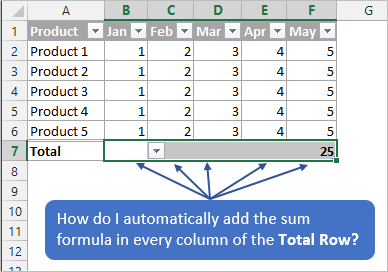How do I automatically add the sum to all the columns in the Total Row of the Table?Don

## The Problem

Unfortunately, there is no built-in way to do this in Excel.  When you click the Total Row checkbox in the Table Tools ribbon, it only adds the SUBTOTAL formula to the bottom right corner of the Excel Table.

To compound that problem, when you copy/paste this formula to the other cells in the Total Row, the results are incorrect.  This happens because the structured column reference (column names) do NOT change within the formula.  All the pasted formulas will still reference the column of the copied cell.In the video below I explain a few solutions to this problem.  You can see read the explanations below the video.

## Solution #1 – Drag Formulas Across

Instead of copy/paste, you can drag the formulas across the cells to get the correct results.  Dragging the formulas will change the column reference in the formula.  This behaves more like the relative references you are used to when working with formulas that contain cell references (B2:B5).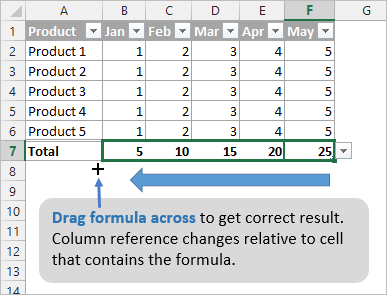This requirement to drag a formula across instead of copy/paste, is just a weird characteristic of a Table.  It's good to understand how this works because it will help you in a lot of situations when you are using structured references (formulas that reference column names instead of cell addresses).

The general rules for structure reference formulas:

• Dragging the formulas across makes the references behave as though they were relative references (B2:B5).
• Copying & pasting the formulas across makes the reference behave like absolute references (\$B\$2:\$B\$5).

Checkout my other article on Absolute Structured References in Excel Table Formulas for more details on this behavior.  I also have a free add-in that will help you convert your formulas between absolute and relative references.

## Solution #2 – Use AutoSum to Quickly Create Subtotal Formulas

The AutoSum feature can be used to create the SUBTOTAL formulas in the Total Row.

1. Simply select the cells in the Total Row that you want to create sum formulas for.
2. Then press the AutoSum button in the Home tab of the ribbon.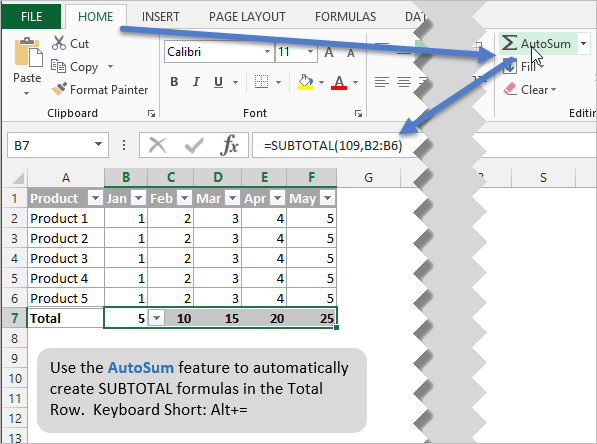This is a very fast and easy solution because it does NOT require the mouse.  Instead, you can use the keyboard shortcut Alt+= (hold down Alt, then press the equal sign key).

If you have a table with lots of columns, this will be much faster than dragging the formula across with the mouse.  This is especially true if you are using a laptop trackpad for the mouse.

The one drawback to this method is that AutoSum does not use structured referencing.  That means it creates a formula that contains the cell references instead of column references.  For example, AutoSum will create a formula that looks like this =SUBTOTAL(109,B2:B6).  It contains the cell addresses instead of the column name.

This might actually be an advantage because you could then use copy/paste to paste this formula to other cells in the Total Row.

## Bonus Tip

The keyboard shortcut to turn the Total Row on/off is Ctrl+Shift+T.  You must have a cell selected in any part of the Table for this to work.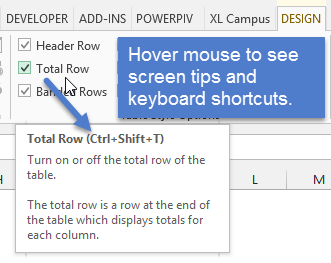If you are new to Tables, checkout my full video tutorial on Excel Tables that will explain all the benefits and features of Tables.  Even though there a few quirks and limitations, Excel Tables are awesome and will save you a lot of time!

Learn how create Absolute Structured References in Excel Table Formulas.

A whole book on Tables! My friends Zack Barresse and Kevin Jones have written THE GUIDE to everything Tables.  This is a great read if you are looking to master Excel Tables. It also includes tons of information and resources for writing VBA for Tables.
eBook Version – Excel Tables Book
Paperback version – Excel Tables BookYou can submit your Excel or VBA question here and I will do my best to get it answered.  Don't forget to subscribe to my free email newsletter below to stay updated.

Do you have another way to create the SUBTOTAL formulas in a Table?  Please leave a comment below with any questions or suggestions.

•Don says:

Jon-
this is a great tip! I could not find anyone with a solution to adding totals across multiple columns in a table so these are great solutions. keep up the great work!

•Jon Acampora says:

Thank you Don! I’m glad to hear it helped, and that you are using Tables. I just added a link to a book my friends wrote about Excel Tables. It’s a great resource that includes a lot of VBA code for Tables.

•S.GUNAA says:

how to change total row,%,
how to add rounded off + or –

•Rajkumar says:

How many cells in computer

•Shafaq says:

Hi,
The total Row option in excel tables provides the sum of columns (total of each column appears at the bottom of the table. what if someone needs the sum of each row in a table at the right side of the table

•Jon Acampora says:

Hi Shafaq,
we can use a SUM formula and the AutoSum feature for this. First, select the cell directly to the right of the Table. This will be the first cell in the data row, not the header row. Then press Alt+= on the keyboard. Hold the Alt key, then press =. A SUM formula will be created. Press Enter to fill the formula down all rows of the Table.

• […] 10. Sum All Columns in The Total Row of an Excel Table + Video […]

Generic filters
Exact matches only

#### Excel Shortcuts List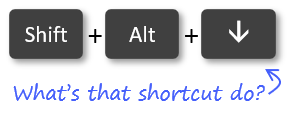Learn over 270 Excel keyboard & mouse shortcuts for Windows & Mac.

Excel Shortcuts List